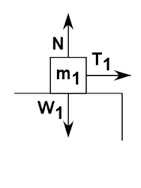## Atwood Machine on a Frictionless Table

In this type of problem, the pulley system is set on the edge of the table with one mass on the table being pulled and the other hanging mass providing the force for the motion. The diagram would look like this:For this case, F=ma still applies and the shortcut equation used on the previous page can be used to find the accelertion of the system. Only m2 is providing the force to accelerate the system.

Since Fnet = ma,

then Fnet = (m1+ m2)a

and a = Fnet/(m1+ m2)

a = (m2g)/(m1 + m2)

To solve for the tension connecting string, the single body system must be used for each mass:The free body diagram above is for the mass on the table. Notice the three vectors drawn for the forces acting on it.

The equation for this mass is:

Fnet = ma

Right – Left = ma

T = m1a

*Choose the direction of motion to the right for m1 and therefore down for m2.

The free body diagram below is for m2:The equation for this mass is:

Fnet = ma

Top – Bottom = ma

T – W2 = m2(-a)

T – m2g = m2(-a)

T = m2g – m2a

OR

T = m2(g – a)

Now, the two equations can be used to solve for T, OR this method can be used by setting the tension equations equal to each other and solving for acceleration.

(source)